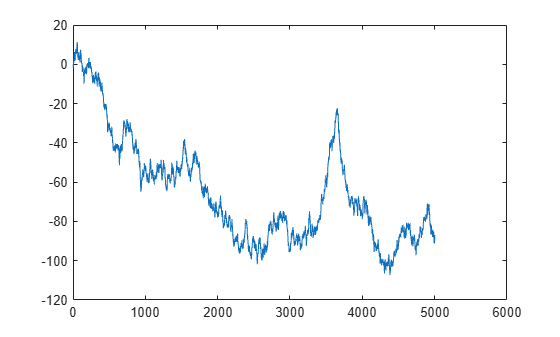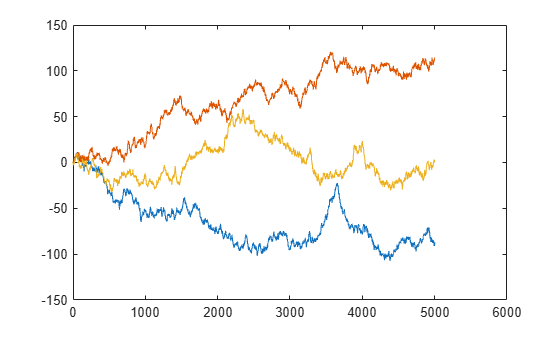# RandStream.create

Create statistically independent random number streams

## Syntax

``s = RandStream.create(gentype)``
``[s1,s2,...] = RandStream.create(gentype,'NumStreams',n)``
``[___] = RandStream.create(gentype,Name,Value)``

## Description

``` `s = RandStream.create(gentype)` creates a single random stream that uses the uniform pseudorandom number generator algorithm specified by `gentype`. `RandStream.list` returns all possible values for `gentype`, or see Creating and Controlling a Random Number Stream for details on generator algorithms. The `RandStream` function is a more concise alternative when you need to create a single stream.```

example

``` `[s1,s2,...] = RandStream.create(gentype,'NumStreams',n)` creates `n` random number streams. The streams are independent in a pseudorandom sense. The streams are not necessarily independent from streams created at other times. NoteNot all generator types support multiple streams. Use either the multiplicative lagged Fibonacci generator (`'mlfg6331_64'`) or the combined multiple recursive generator (`'mrg32k3a'`) to create multiple streams. ```

example

````[___] = RandStream.create(gentype,Name,Value)` controls creation of the stream using one or more `Name,Value` pairs.```

## Examples

collapse all

Create three independent streams. Generate random numbers from each stream. Check the correlations between them. The correlations between different streams are not exactly 0 because they are calculated from a sampling of the distribution.

```[s1,s2,s3] = RandStream.create('mrg32k3a','NumStreams',3); r1 = rand(s1,100000,1); r2 = rand(s2,100000,1); r3 = rand(s3,100000,1); corrcoef([r1,r2,r3])```
```ans = 3×3 1.0000 0.0016 -0.0019 0.0016 1.0000 -0.0012 -0.0019 -0.0012 1.0000 ```

You can also create one stream from three independent streams and designate it as the global stream.

```s2 = RandStream.create('mrg32k3a','NumStreams',3,'StreamIndices',2); RandStream.setGlobalStream(s2);```

Generate random numbers from the global stream.

`r = rand(100000,1);`

Create three mutually independent streams to simulate one-dimensional random walks using the method `RandStream.create`.

`[s1,s2,s3] = RandStream.create('mrg32k3a','NumStreams',3);`

Generate a random walk from the first stream. First, set it as the global stream. Use the first stream to generate 5,000 random steps from the standard normal distribution. Choose the starting position at 0 and use `cumsum` to calculate the cumulative sum of the random steps. Plot the resulting random walk.

```RandStream.setGlobalStream(s1) dy1 = randn(5000,1); y1 = cumsum([0; dy1]); plot(y1)```Repeat the process using the second and third streams. Plot the results on the same axes.

```hold on; dy2 = randn(s2,5000,1); y2 = cumsum([0; dy2]); plot(y2) dy3 = randn(s3,5000,1); y3 = cumsum([0; dy3]); plot(y3) hold off```Calculate the correlations among the streams. The correlations between different streams are not exactly 0 because they are calculated from a sampling of the distribution.

`C = corrcoef([dy1 dy2 dy3])`
```C = 3×3 1.0000 -0.0363 0.0155 -0.0363 1.0000 -0.0012 0.0155 -0.0012 1.0000 ```

## Input Arguments

collapse all

Random number generator algorithm, specified as a character vector or string scalar naming a random number generator. MATLAB® offers several generator algorithms. The following table summarizes the names and key properties of the available generator algorithms. For more information, see Creating and Controlling a Random Number Stream.

NameGeneratorMultiple Stream and Substream SupportApproximate Period In Full Precision
`'mt19937ar'`Mersenne Twister (used by default stream at MATLAB startup)No219937-1
`'dsfmt19937'`SIMD-Oriented Fast Mersenne Twister No219937-1
`'mlfg6331_64'`Multiplicative lagged Fibonacci generatorYes2124 (251 streams of length 272)
`'mrg32k3a'`Combined multiple recursive generatorYes2191 (263 streams of length 2127)
`'philox4x32_10'`Philox 4x32 generator with 10 roundsYes2193 (264 streams of length 2129)
`'threefry4x64_20'`Threefry 4x64 generator with 20 roundsYes2514 (2256 streams of length 2258)
`'shr3cong'`Shift-register generator summed with linear congruential generatorNo264
`'swb2712'`Modified subtract with borrow generatorNo21492
`'mcg16807'`Multiplicative congruential generatorNo231-2

### Name-Value Pair Arguments

Specify optional comma-separated pairs of `Name,Value` arguments. `Name` is the argument name and `Value` is the corresponding value. `Name` must appear inside quotes. You can specify several name and value pair arguments in any order as `Name1,Value1,...,NameN,ValueN`.

Example: `RandStream.create('mrg32k3a','NumStreams',3,'StreamIndices',2)`

Number of independent streams to create, specified as the comma-separated pair consisting of `'NumStreams'` and a positive integer.

Stream indices, specified as the comma-separated pair consisting of `'StreamIndices'` and a vector of positive integers or a positive integer. Specify this parameter to index the current stream from among the group of streams with which it was created. The default value is `1:N`, where `N` is the value of `'NumStreams'`.

Random number seed, specified as the comma-separated pair consisting of `'Seed'` and a nonnegative integer or `'shuffle'`. The seed specifies the starting point for the algorithm to generate random numbers. `'shuffle'` creates a seed based on the current time. If you specify an integer, it must be between 0 and 232 − 1.

Specify the generator seed as an initialization step when creating a stream at MATLAB startup or before running a simulation. To reproduce a stream, use the same seed every time. While using multiple seeds will create multiple sequences of random numbers, there is no guarantee that the different sequences are statistically independent. In situations where this is important, use `RandStream.create` with multiple outputs to create multiple streams that are statistically independent.

Transformation algorithm to generate normally distributed random numbers from the random number stream using `randn`, specified as the comma-separated pair consisting of `'NormalTransform'` and one of the algorithm names `'Ziggurat'`,`'Polar'`, or `'Inversion'`.

Option to return cell array, specified as the comma-separated pair `'CellOutput'` and logical `false` (`0`) or `true` (`1`). If you specify `'CellOutput'` as `true`, `RandStream.create` returns the stream objects as elements of a cell array.

## Tips

Typically, you call `RandStream.create` once to create multiple independent streams in a single pass or at the beginning of a MATLAB session. For example, you can create three independent streams by using `[s1,s2,s3] = RandStream.create('mrg32k3a','NumStreams',3)`.

Alternatively, you can create each stream from a separate call to `RandStream.create`, but you must specify the appropriate values for `gentype`, `'NumStreams'`, `'Seed'`, and `'StreamIndices'` to ensure their independence:

• Specify the same values for `gentype`, `'NumStreams'`, and `'Seed'` in each case.

• Specify a different value for `'StreamIndices'` each time. All values should be between `1` and the value of `'NumStreams'`.

For example, create two independent streams by using ```s1 = RandStream.create('mrg32k3a','NumStreams',5,'Seed',0,'StreamIndices',1)``` and ```s2 = RandStream.create('mrg32k3a','NumStreams',5,'Seed',0,'StreamIndices',2)```.